`The Greatest Misconception in Science: Speeds Greater Than the Speed of Light in an Expanding Universe`
|   Home   |   Books   |   Reports   |   Reviews   |   Contact Us   |   Press Releases   |   Submissions   |

## The Greatest Misconception in Science: Speeds Greater Than the Speed of Light in an Expanding Universe

### Introduction

It is firmly established in special relativity that bodies cannot travel faster than the speed of light. Indeed, to cause an object to travel ever closer to the speed of light requires an ever increasing amount of energy. In special relativity, this follows from the formula relating the energy E of an object of mass m to its speed v:

E = mc2/√1 - (v/c)2            (1)

where c is the symbol for the speed of light, which has a value of 299,792,458 meters per second. For a body to achieved the speed of light, an infinity amount of energy is required, which is another way of saying that it cannot happen. Mathematically, as v approaches c, (v/c) approaches 1, and E becomes arbitrarily large as the denomination in Eq.(1) becomes arbitrarily small. By the way, the famous equation E = mc2 corresponds to the case when the object is not moving and v = 0 in Eq.(1).

In general relativity, space and time are dynamic. Space can bend, twist and stretch. Albert Einstein developed general relativity as a theory of gravity that is locally compatible with special relativity. The force of gravity is a consequence of the curvature of space.

In the standard model of cosmology, space has been stretching since the beginning of time. Many cosmologists believe that distant space points can move apart from one another faster than the speed of light. This common belief can be found in textbooks,1 articles,2 and Internet pages including those of Wikipedia.3 This would seem to be incompatible with special relativity, but those cosmologists who believe in supraluminal speeds (v > c) say that space is different from an object. In other words, distant space points can move apart faster than c because they are not physical objects.

However, I shall argue that such supraluminal speeds are not possible because (i) general relativity is compatible with special relativity and (ii) small objects could be placed at the two space points of interest, in which case, the objects would travel apart from one another faster than the speed of light.

What is the source of this confusion? The expansion of the Universe (that is, the stretching of space) creates some ambiguities in determining distances and hence speeds. When an object moves a distance Δd during a time interval Δt, its speed is, by definition, the former divided by the latter:

v = Δdt            (2)

It is the difficulty in defining distance in an expanding Universe that has led to the belief among many cosmologists that a space point sufficiently far away from Earth can recede faster than the speed of light. In the rest of this report, we shall elaborate on the statements in this Introduction, and, in the Conclusion, we shall explain what has gone wrong with the thinking of some scientists.

### Expanding Space and Hubble's Law

It is well established that the Universe is expanding. More precisely, in each time interval, space stretches by a small amount thereby effectively “pushing” objects apart. This is not noticeable on small distance scales such as on Earth, in the solar system or even within the Milky Way Galaxy. However, it is evident for faraway objects such as galaxies and quasars. If space is expanding then distant galaxies should all be moving away from us independent of the direction in which we look. This indeed was observed by Edwin Hubble in 1929 using a telescope at the Mount Wilson Observatory.

The speed vrec that a faraway object recedes from Earth is given by Hubble's Law:

vrec = H(t)dprop            (3)

where dprop is the proper (or co-moving) distance and H(t) is know as Hubble's constant. As indicated, it is function of time t and is not actually a constant. However, H(t) varies slowly with time and, one would have to wait for hundreds of millions years to observe a significant change in its value. In what follows, we shall treat H as constant and equal to its current value H0, which is approximately 68.5 kilometers per second per megaparsec. A megaparsec is a unit of distance equivalent to about 3.1 ten-billion-trillion meters (3.1 x 1022 meters) or about 3.26 million light-years.

Hubble's law means that the further a galaxy is from us, the faster it moves away from us. Here is the picture: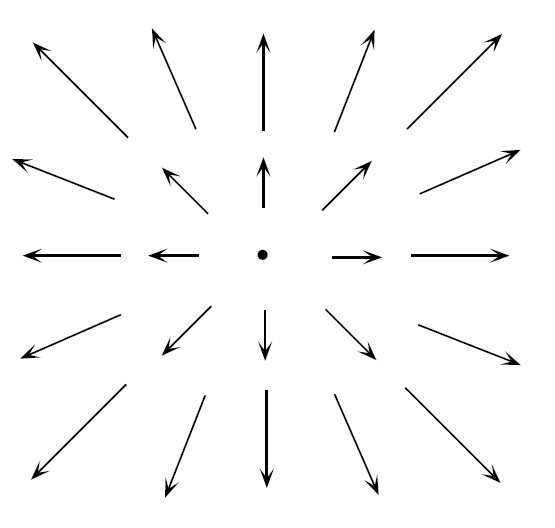Figure 1. Illustration of Hubble's Law

The Earth is located at the center of Figure 1, and the arrows represent the velocities of faraway galaxies.

The expansion of the Universe can be visualized as follows. Consider the following grid of space points: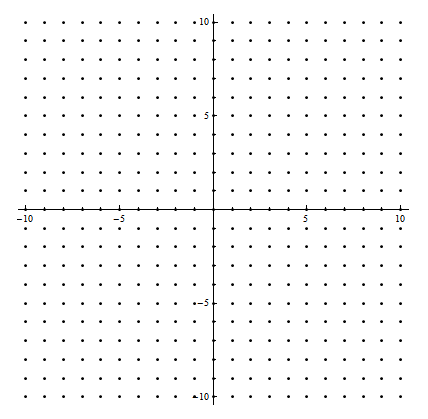Figure 2a. A Grid of Points in Space

After some time has elapsed, space will stretch and move the points apart leading to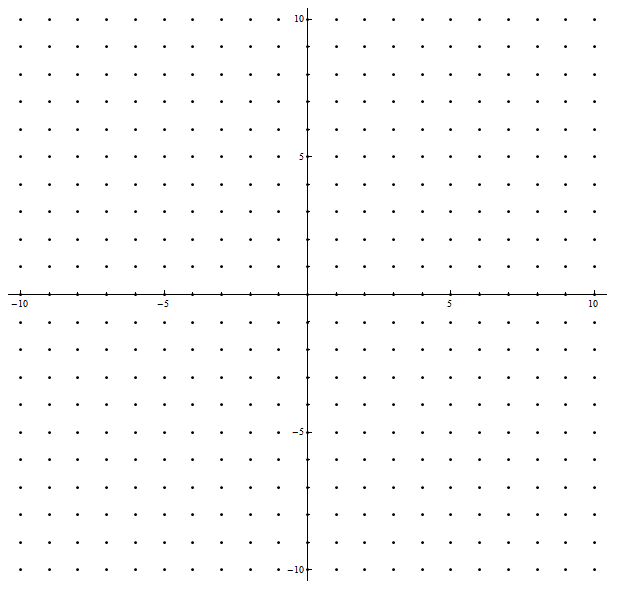Figure 2b. The Grid of Points in Space of Figure 2a at a Later Time

Note that from the point of view of a person at the origin, all points move away. However, this is also true of any other point in the above grid. Everything is moving away from everything else. Hence, if there was an observer on a faraway planet in a faraway galaxy, distance galaxies would still appear to be moving away from that observer. It would be as in Figures 1 and 2 with that observer at the center point.

Equation (3) is just a consequence of the definition of the stretching of space. Each interval of space stretches by the same factor. Consider the following, which represents what happens in one direction.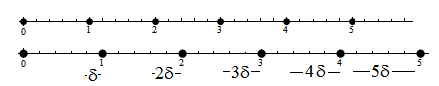Figure 3. Derivation of Hubble's Law

The top line is the situation at a certain time t and the bottom line is at a later time t + Δt. During the time interval Δt, point 1 moves a distance δ to the right. The distance between points 1 and 2 also increases by δ so that when it is added to the increase between the points 0 and 1 (which is also δ), one gets an increase of 2δ between 0 and 2. Likewise, the distance between 2 and 3 increases by δ, and when added to the increase between 0 and 2 (which is 2δ), one obtains 3δ for the increase between 0 and 3. And so on. At the distance d = nd0 (where d0 is the distance between two consecutive points in Figure 1), the increase is nδ. Since the speed v of the nth point is by definition the change in distance divided by the time interval, one gets v = nδ/Δt = (dδ)/(d0Δt), which is Hubble's law in Eq.(3) if one identifies H with δ/(d0Δt).

### Why Some Cosmologists Believe in Supraluminary Speeds in an Expanding Universe

According to Eq.(3), the recessional speed of distant objects increases without limit. Hence, there is some distance at which point vrec = c. This distance, which is known as the radius of the Hubble sphere, is easily seen from Eq.(3) to be c/H0. Beyond this distance, vrec > c. This is the basis for why many cosmologists believe in recessional speeds faster than the speed of light for very distant objects.

In addition, it can be shown that light emitted from very, very far away galaxies never reaches the Earth (as long as the Hubble constant remains non-zero). The light does not reach us because space expands sufficiently fast as to carry the light away from us. This also would seem to suggest that distant space is moving faster than the speed of light.

### What is Wrong with the Argument in the Previous Section

The first issue with using vrec (in Eq.(3)) as the speed of a faraway object is that it is based on the proper distance dprop. It turns out that there are other ways of defining the distance to a faraway object. Commonly used ones are: the luminosity distance (which is determined by the apparent brightness of the object if the absolute brightness is known), the parallax distance (which is determined by seeing how the apparent position of the object changes against very distant objects as the Earth moves around the Sun), the angular size distance (which is obtained by measuring the apparent width if one knows the absolute width of the object), and the time-of-flight distance (which is the distance light traverses as it goes from the object to Earth, or equivalently the time it takes the light to travel this distance times c).

If the Universe is not expanding, all these definitions produce exactly the same distance. Even when space is stretching, they give values very, very close to one another for short distances. However, for very faraway objects, they differ significantly. When a speed is computed as the change in distance divided by the change in time for each of these different definitions of distance, one obtains different speeds. So the question arises as to which speed should one use? I shall argue that vrec is not a good definition of speed for faraway objects.

This may sound silly but suppose someone defines his definition of distance as twice the ordinary definition of distance. Then, when the ordinary speed becomes greater than c/2, this “silly” definition producs a speed more than c. This does not mean that objects travel faster than the speed of light; it means that a silly definition of distance has been chosen. In some sense, for objects very faraway, vrec differs from a “good” definition of speed by factors greater than 1.

The proper distance differs from the other above-mentioned distances in that it is “instantaneous.” The other definitions involve a time interval, which in most cases, is close to the time it takes light to travel from the object to Earth. During this time interval, space stretches. Hence, the distance is varying and the above definitions are in some rough sense an average distance between the object and Earth during the time interval in which the measurement is being made. To drive home this point, consider another definition of distance, which I call the “rocket-ship” definition: A rocket is sent from the object to Earth. Along the way, a “being” in the rocket measures distances and adds them up to obtain the total distance. Then, this rocket-ship distance depends on the speed of the rocket. If the rocket moves slowly, space will stretch more during the trip and the rocket-ship distance will be greater than if the rocket sped along more quickly.

A problem with using the proper distance dprop is that it involves an instantaneous non-local measurement. Such measurements are not possible and violate the fundamental principle of causality. One cannot just sit “outside the Universe”, look at it as one does in Figure 2, and measure dprop. The proper distance can be theoretically computed if one knows a priori the exact nature of the stretching of space. However, imagine a situation in which different regions of the Universe are stretching by different unknown factors (a situation in which the Hubble constant varies with location: H = H(x,t)) so that one would have to measure instantaneously all the “varying” stretchings of space between Earth and the object to obtain the proper distance. Then, it would not be possible for someone on Earth to calculate the proper distance.

The best that one could do is to arrange a “conspiracy” of multiple observers between the object and Earth. Observers are placed at relatively short distance intervals all the way from Earth to the object. At an agreed upon time, which may actually be difficult to implement in practice if the stretching of space varies locally, they measure the distance to and the speed of the successive observer. They all wait an agreed upon time interval and repeat the measurements. They then radio all this information to an observer on Earth who uses it to compute the proper distances at the two times of the “experiment”. If the person on Earth simply sums up all the distances for the first measurement, he or she will get the proper distance dprop. At the later time, when the measurements are repeated, dprop is greater, and a speed using Eq.(2) can be computed. The result will agree with Eq.(3).

However, since general relativity is locally compatible with special relativity, one should not naively sum the distances. When two observers are moving relative to one another (and this is the case for all the interpolating observers), there are formulas in special relativity for adding distances and combining velocities. If one uses these formulas then the computed distance is less than dprop because of Lorentz contractions. When the correct combining of distances is performed using special relativity and the speed is computed, it is always less than the speed of light c. Alternatively, the formula in special relatively for combining velocities can be used. It produces a speed for the object, which agrees with the one obtained by combining the distances correctly and not naively.

### The Redshift

Light emitted from an object moving away from an observer is redshifted, meaning that the frequency is lower than would be the case if the object were at rest. Since wavelength and frequency are inversely related, the wavelength is increased for electromagnetic radiation emitted by a receding body. The word “redshift” is used because red is in the lower frequency part of the visible-light spectral region. Hence, if an object is moving fast enough, ordinary light or even blue light will become red light. This type of redshift is known as the Doppler effect.

It is physically straightforward to see why the redshift occurs. Suppose a wave is created by some physical process such as the moving of electrons up and down in an antenna in the case of (electromagnetic) radio waves or the pushing of a buoy up and down in the case of water waves. If the emitter of the waves is moving away from the observer then between two down beats the object increases its distance from the observer. If the speed of the wave is constant, that is, independent of the motion of the emitter, which is the case for most waves including electromagnetic waves, then the trough associated with the second beat takes longer (compared to a motionless emitter) to arrive at the observer because of the “extra distance” that is created and must be traversed. Hence, the frequency, which is the time between down beats, decreases. In a similar manner, the wavelength, which is the length between two down beats, or troughs, of the wave, is increased by the extra distance created by the motion of the receding emitter during the two beats. This is illustrated well in the figures and the animations at the Wikipedia page on the Doppler effect.

A redshift is observationally seen for faraway galaxies. Since faraway galaxies are moving away from Earth, this is not surprising. However, in an expanding Universe, cosmologists typically attribute the redshift to the stretching of space and not to a Doppler effect. The wavelength of light is stretched by the same factor that space is stretched. When the wavelength of an electromagnetic wave is increased, its frequency goes down. This type of redshift is called the cosmological redshift.

### A More “Natural” Definition of Cosmological Distance

In an article by the author of this report,4 a quite natural definition of the speed of a distant object was proposed. As above, one sets us a series of observers lined up between the distant object and Earth. A sufficiently large number of observers is used so that measurements in a neighboring observer's region can essentially be considered local.

The observer closest to the object notes the object's speed and distance and sends a light signal toward Earth with this information. The observer second closest to the object notes the speed of and distance to the closest observer just as the signal from the closest observer passes the second-closest observer and sends this information to Earth in another light signal. This continues with the third closest observer sending the information about the second closest observer and so on. A person on Earth then receives all the electromagnetic signals from all the observers at the same time and the information contained in them. See Figure 4. Then, the special-relativity formula for combining the speeds is used to provide a speed for the distant object, which we call vphys, suggesting that we consider it to be more “physical” than other definitions. The value of vphys is guaranteed to be less than the speed of light because of the use of special relativity formulas. Distances can also be combined using formulas from special relativity to obtain a “physical” distance dphys, and a computation of speed based on using Eq.(2) would also produce vphys when Δt is also determined using special relativity.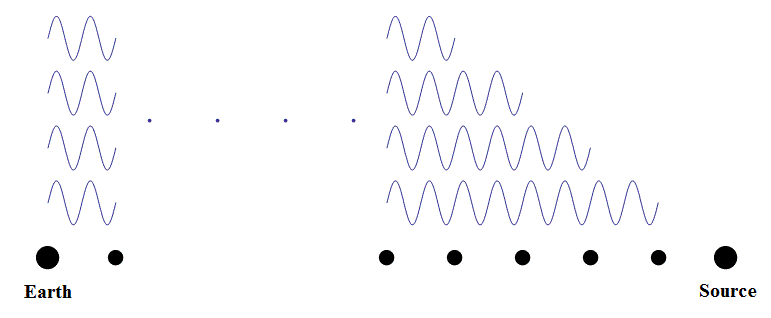Figure 4. Construction of a Physical Distance and Speed
The medium sized dots represent the location of the interpolating observers.
The light signal from the observer closest to the source is the wave located lowest in the figure.
The light signal from the second closest observer is the wave above the first one, and so on.
These waves are drawn next to one another (rather than on top on one another) for “clarity.”

Note that only local measurements are used to obtain the distant object's speed vphys, and during the measuring process, there is no violation of causality at any stage: The information is conveyed to Earth using electromagnetic waves.

What makes this definition of speed interesting is the following: It can be shown4 that the value of the cosmological redshift is the same value as the redshift computed by the relativistic Doppler formula using vphys as the recessional speed. This agreement occurs even if the distant object has what is know as a peculiar velocity, that is, a velocity not entirely attributable to the expansion of the Universe. Agreement is also obtained for any time dependence in the Hubble constant and even if the stretching of space varies spatially, that is, from place to place between the distant object and Earth.

It is sometimes stated that the cosmological redshift cannot be computed as a Doppler effect. The argument goes as follows. Suppose the Universe is not expanding at the time that a distant object emits its light in the direction of the Earth, but begins after that. Also, suppose that just before the light is received on Earth, the expansion of the Universe slows and then stops. Then, one might think that there can be no Doppler redshift because the emitting object and the receiving observer were “still” at the times of emission and reception. However, this argument is not good. Among things, it assumes that the relative speed between two distant objects is instantaneously obtained. How does a person on Earth know that the faraway object is “still” at the time of emission? Indeed, to physically measure the speed of a distant object as is done in the first paragraph of this section requires a time interval. Because of this, the velocity for vphys is built up over time. The fact that the Doppler redshift using vphys as the speed does produce the cosmological redshift demonstrates that the latter can be obtained from the former.

### Beyond the Event Horizon

The observable Universe is that part of the Universe which one can “see” from Earth. If the Universe were static and not expanding, the radius of the observable Universe would be the age of the Universe times the speed of light, which when evaluated is about 13.8 billion light-years. The current proper distance to the edge of the observable Universe is about 46 billion light-years, because the object that emitted the light about 13 billion years ago has moved considerably farther away from Earth in the 13 billion-year interim.

The (proper-distance) radius of the Hubble sphere, c/H0, is 14.2 billion light-years. As explained above, the speed of objects beyond this radius do not travel faster than the speed of light, contrary to what is stated in the scientific world, if a physical definition of speed is used.

The cosmological event horizon is the (proper) distance beyond which light emitted currently can never reach the Earth. This clearly depends on the future expansion of space. If space were to slow its stretching and then stop, then the event horizon would move off to infinity and be eliminated. One might try to argue that points in space beyond the event horizon travel away from Earth faster than the speed of light. The problem with this statement is that since one can never physically observe such points (or astrophysical objects at these points), one really does not know their speed. It is impossible to measure vphys using the method outlined in the section entitled A More “Natural” Definition of Cosmological Distance above. Furthermore, if one were to imagine halting the stretching of space at some future time, all points of space would eventually be observable (since the cosmological horizon becomes infinite) and, using vphys as the definition of speed, one would find that such points do not travel faster than c. Finally, just because an event horizon exists, it does not necessarily mean that an object beyond the horizon travels faster than the speed of light. The constant acceleration of an object illustrates this.

### The Accelerating Object in a Static Universe in Special Relativity

It is useful to consider another situation, the constant acceleration problem. It is somewhat analogous to an expanding Universe but does not involve the stretching of space.

The setup is as follows. A man and a woman start out at the same place at the same time. The woman, an astronaut, gets into a rocket with incredibly powerful engines and takes off. The rocket is able to fly in a straight direction constantly accelerating itself and the woman. We assume that this acceleration continues indefinitely although the amount of energy (and fuel) to conduct this trip is eventually enormous.

As part of the experiment, the two people constantly keep in communication. Thus, the man on the ground continually sends a visible light image of a clock on Earth to the rocket. Similarly the female astronaut sends electromagnetic light waves to man so that he can see what is happening to the rocket.

If the rocket continually accelerates, its speed will forever increase. Eventually, the speed will approach that of light. However, the rocket never achieves that speed. The speed will always be a little less than c.

The man on the ground on Earth sees the rocket zoom off. As the rocket goes faster and faster and approaches the speed of light, two interesting things happen. First, he observes that the nature of the electromagnetic signals from the rocket change: the wavelengths get longer and the frequencies go down. This is the Doppler effect. The light becomes yellowish, then reddish, and then infrared – so the man on the ground has to use an infrared detector to see the rocket. After a long time, even this is not good enough: the electromagnetic signals become microwaves and finally radio waves. Secondly, the astronaut's time seems to slow down increasingly. Indeed, the second hand on a clock fastened to the rocket moves slower and slower and almost stops.

The woman astronaut experiences the acceleration and feels pinned against her seat by a “constant force”. In the “rear view mirror”, she observes the man moving ever increasingly farther away. She, also, has the same two interesting experiences (as the man in the previous paragraph) concerning the electromagnetic signals being sent to her from Earth: the wavelengths get longer and the frequencies go down while the nature of the signals evolve through the spectrum from red all the way to radio waves. Likewise, she observes the images of the clock on the ground “ticking time” slower and slower. Indeed, this happens so much that the time on the clock never surpasses a certain limiting time, TL. This does not happen for the man observing the rocket. It is possible to observe the entire future of the rocket, although the man on the ground might have to wait “exponentially” long to see what transpires at very late times.

Even more amazing is that electromagnetic signals sent by the man after the time TL never reach the woman. This phenomenon is known as an (apparent) horizon for the rocket because events on Earth occurring after TL cannot affect the rocket and the astronaut. A name for TL might be a time horizon. The woman has accelerated to such a fantastic speed that electromagnetic waves sent after a certain time never reach her. Does this mean she is moving faster than the speed of light? Everyone who knows special relativity will agree that the answer is no. These electromagnetic waves do not reach her because of the “head start” she has over them and because her speed is very close to c and ever increasing.

So, if a horizon exists, it does not necessarily mean that objects on the other side of the horizon travel faster than the speed of light. The constant acceleration situation is an example.

Here is a diagram that represents the situation: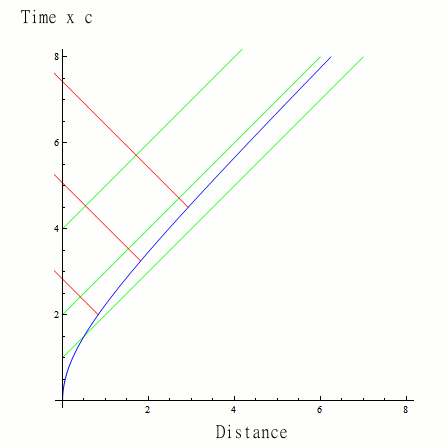Figure 5. The Space-time Diagram for the Constant Acceleration Situation

In the figure, which is called a space-time diagram (for the observer on Earth), the horizontal axis is the distance the rocket has traveled. Let us select distance units in which 1 corresponds to a light-year as measured by the man on Earth. The vertical axis is the the speed of light times the time (in years) elapsed since the rocket took off as measured by the man. When one multiplies time by c, the units become that of distance and hence the y- and x-axes have the same units. When the distance is measured in light-years as we are doing, then 1 on the vertical axis corresponds to 1 year of elapsed time. The man, who does not move, remains at a distance of zero; his trajectory is the y-axis in Figure 5. The rocket, which constantly accelerates to the right, traces out the blue curve in Figure 5. At time zero, both the man and the women are at the same location, which is represented by the origin in the diagram.

A trajectory of a light ray emitted in the direction of the rocket is a straight line with a slope of 1 (45 degrees) in Figure 5 precisely because the units for the vertical axis are time x c. Figure 5 displays some of the trajectories of the light sent by the man to the woman; They are the green lines. On the other hand, a trajectory for a light ray sent from the rocket to the man is a straight line with a slope of -1 (45 degrees to the left). Some of the electromagnetic signals sent from the rocket to the man are displayed in red in Figure 5.

Because the slope of the blue curve approaches 1, the speed of the rocket eventually is very close to c. Notice that the trajectories for electromagnetic signals send from Earth to the rocket do not intersect the rocket's trajectory for times greater than 2/c (for the particular acceleration we have selected). In other words, for the case represented in Figure 5, cTL = 2. The woman will never see the clock on Earth having a time greater than 2/c x (a light year) or two years for the units that we are using. On the other hand, all electromagnetic signals sent from the rocket (the red lines in Figure 5) eventually reach the man (his location corresponds to origin with Distance = 0). This explains the above statement “It is possible to observe the entire future of the rocket [through the signals represented in red in Figure 5], although the man on the ground might have to wait 'exponentially' long to see what transpires at very late times.”

Although the man and the women see the other one moving away at the same accelerating rate, only the woman is subjected to a force. Her situation is special and is associated with a non-inertial reference frame. In an inertial frame, a person, by definition, experiences no forces. In outer space, where gravity is absent, an inertial reference frame is one at rest or moving with a constant speed. Although a person on the surface of the Earth is not moving, that person's reference frame is technically speaking not inertial because the person feels the force of gravity. Albert Einstein realized that, at the surface of the Earth, a person undergoing free fall (such as in the first part of a bungee jump (ignoring air friction)) is associated with an inertial reference frame: such a person would not feel the force of gravity (or any other force in the absence of air friction). However, the man on the Earth is not accelerating because gravity is counterbalanced by a support force under his feet. In addition, the reference frame associated with the man is almost inertial and does not lead to significant effects, e.g., only an extremely small gravitational redshift and time delay.

In the above “rocket experiment,” what happens if the constant acceleration ends. As the rocket decelerates, the electromagnetic waves sent by the man and observed by the woman astronaut cease being lengthened any further. Similarly, their frequency stops declining. The man observes the clock on the rocket to tick slowly but there is no longer any additional slowing of its rate. The woman observes exactly the same thing as the man. Even though the women is moving close to the speed of light, her observations of the clock on Earth indicate that time is moving slowly, but steadily, and thus the limiting time is eliminated. If she lived long enough, she would be able to observe the entire future of the man on Earth through the images being sent to her from him. In terms of Figure 5, the blue line would “stop curving” and achieve a constant slope slightly larger than 1 (or 45 degrees). Then, any light (a green line in Figure 5) associated with an electromagnetic propagation toward the rocket would eventually reach the woman. In other words, a limiting time TL no longer exists.

The situation in an expanding Universe is analogous to the above. Although a distant object and an observer on Earth are essentially both inertial observers on the scale at which we are considering things, a distant object appears to accelerate away from us because the farther away it gets, the higher its speed. Therefore, the cosmological event horizon can be considered analogous to the apparent horizon associated with an accelerating object. Hence, one cannot necessarily conclude that a very faraway object is traveling faster than the speed of light just because an electromagnetic signal sent from it will never arrive on Earth. The reason can be the same as for the constant acceleration situation: from the point of view of the far away object, we have a “head start” on the electromagnetic waves coming from the object, and we are continually accelerating to maintain a lead over them.

### Conclusion: What Has Gone Wrong with Cosmologists' Thinking

Albert Einstein is famous for many things. But one thing he is famous for that the public generally does not know about is that he is the “father” of the Gedanken or thought experiment. Although he was not the first person to use this method, he used it more effectively and extensively than any predecessor. His development of special relativity proceeded largely through Gedanken experiments and relied just a little on real experimental results. He continued using such methods to develop general relativity and to think about issues in quantum mechanics. In short, Einstein was the master of the use of the Gedanken experiment imagining things that could not be carried out during his time.

Modern theorists often employ thought experiments in their research. One of the key points, however, is that the Gedanken experiment must be physically realizable. It is reported that in his youth, Einstein tried to imagine what it would be like to ride a beam on light. Later in his life, he realized that this was not possible. So this early thought experiment of Einstein was flawed. Nevertheless, it was helpful to him because he realized that it was impossible.

When cosmologists say that two distant spacial points move apart from one another faster than the speed of light, they are performing a Gedanken experiment because they are imagining that they can view the Universe as if they were outside it and can measure everything instantaneously. It is as though cosmologists are God with powerful measuring capabilities.

The cosmologist draws a grid on a piece of paper and imagines all the squares expanding as in Figure 2. Then it is obvious that distance points move apart from one another faster and faster without limit. So two point sufficiently far away from one another must separate from one another faster than the speed of light. The problem with this thinking is that it involves non-local instantaneously measurements in violation of causality and the special theory of relativity because information is communicated faster than the speed of light. Even in the case of a real physical piece of paper lying on a person's desk, it takes time for light to reflect off the paper and travel to a person's eye. The observation is not made instantaneously but at a tiny, tiny fraction of a second in the past. It is also not non-locally made. The light rays from the paper must travel to the person's eyes, and hence to the same spot before the information about the different points on the paper is collected to determine distances on the paper. The measurement is actually made locally.

The brain is a powerful instrument and can imagine almost anything. We can imagine objects that defy gravity and rise instead of sink. We can picture the entire Universe in our minds. And we can imagine objects traveling faster than the speed of light but that does not mean that such objects exist.

References

1 P. J. E. Peebles, Physical Cosmology, (Princeton University Press, 1971), pp. 3-18, 159-189;
Principles of Physical Cosmology, (Princeton University Press, Princeton, 1993), pp. 58-108.

2 W. M. Stuckey, Am. J. Physics 60, 142-146 (1992).
H. S. Murdoch, The Quart. J. R. Astronom. Soc. 18, 242-247 (1977).
T.M. Davis, C.H. Lineweaver, Expanding Confusion: Common Misconceptions of Cosmological Horizons and the Superluminal Expansion of the Universe, Publications of the Astronomical Society of Australia 21, 97–109 (2004).
Dave Rothstein, Is the universe expanding faster than the speed of light?.

3 Wikipedia pages entitled Faster-than-light, Hubble Volume, Hubble's law, Metric Expansion of Space, Comoving Distance, Cosmological Inflation, Observable Universe, Theory of Relativity, Physical Cosmology, Dark Energy, and The Whole Shebang

4 Stuart Samuel, Comments on an Expanding Universe, SLAC-PUB-8747, LBNL-51153 (April 2003).

This report was prepared by Jupiter Scientific, an organization devoted to the promotion of science through books, the internet and other means of communication.

This web page may NOT be copied onto other web sites, but other sites may link to this page.